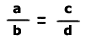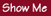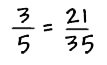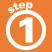Home    |    Teacher    |    Parents    |    Glossary    |    About UsA proportion is a name we give to a statement that two ratios are equal. It can be written in two ways:

• two equal fractions,• or,

• using a colon,    a:b = c:d

When two ratios are equal, then the cross products of the ratios are equal.

That is, for the proportion, a:b = c:d ,  a x d = b xHomework Help | Pre-Algebra | Ratios and ProportionsEmail this page to a friendSearch·  Ratios·  Proportions·  Distance, rate     and time·  Similar figuresFirst Glance In Depth Examples WorkoutProportions